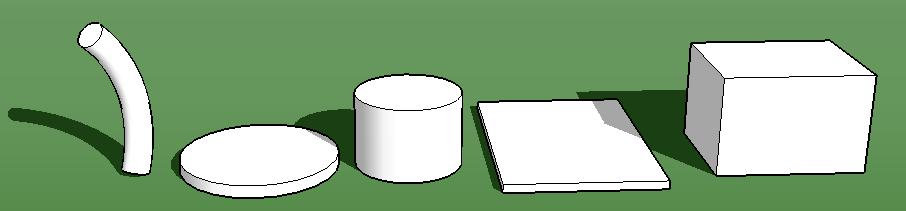SEARCH HOMEMath Central Quandaries & QueriesVolume Calculator We get many questions asking us to calculate the volume of topsoil, gravel, water, concrete, etc. for rectangular and cylindrical (round) shapes. The following tool will help you find the volume for some common shapes.Sorry, Javascript must be enabled on your browser to use this tool.

Turn on javascript and then press Reload to try again.

Which shape most closely resembles yours? Click on it.Cylinder: Round part and length or depth part. Examples: pools, posts, pipes, pies, pizzas, hoses, round gardens and flower beds. Box-shaped: a length, depth and width. Examples: topsoil, concrete, gravel, cement, fill, aquariums, boxes, storage spaces, truck containers, yards, gardens, driveways.

Cylinder shape.

1. Measure the circle part:
Measurement:
 This is the Radius (distance from center to rim) Diameter (distance across the circle) Circumference (distance around the rim)

2. Measure the depth, height or length:
 Measurement: meters centimeters yards feet inches

3. Click

Cylinder shape.

A cylindrical shape that has description has a volume of:

table here.

Box shape.

You need either:

the length, width and depth of the box shape   - or -    the area of the top and the depth
 Length: meters centimeters yards feet inches Width: meters centimeters yards feet inches Depth: meters centimeters yards feet inches

 Area: square meters square centimeters square yards square feet square inches acres hectares Depth: meters centimeters yards feet inches

Box shape.

A box shape that has description has a volume of:

table here.Math Central is supported by the University of Regina and The Pacific Institute for the Mathematical Sciences.